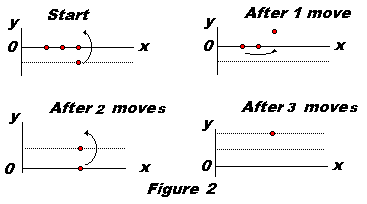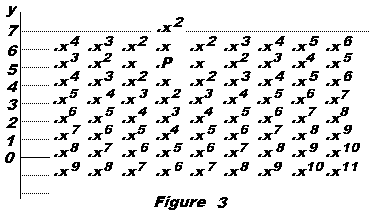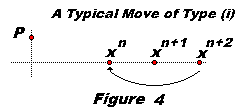The following is an excerpt from
Ross Honsberger,
Mathematical Gems II

## A Problem in Checker-Jumping

### Sending Scouts into the Desert

Everybody knows the leap-frog jump in the game of checkers. There is an interesting problem about checker-jumping on the lattice points of a plane. One begins by arranging a number of men in the starting zone, which consists of the half-plane of lattice points on and below the x-axis. The object is to get a man as far as possible above the x-axis by checker-jumps in the directions of the lattice lines (diagonal jumps are not allowed). You can think of this as sending scouts into the enemy territory (desert). The problem is to determine the least number of men in the starting zone which permits one scout to reach a prescribed penetration depth.

Here is a Java applet to assist you in solving the problem. The desert lies above the horizontal line. Your army is located below. X and Y determine the size of the army. Level is your goal - how far you plan to go into the desert. Try solving the problem for a few first levels before reading further.

(To make a move, click on a chip to be moved and then on the indended new location.)

### This applet requires Sun's Java VM 2 which your browser may perceive as a popup. Which it is not. If you want to see the applet work, visit Sun's website at https://www.java.com/en/download/index.jsp, download and install Java VM and enjoy the applet.

/tr<
 What if applet does not run?1. We see immediately that only two men are needed to reach the first level above the x-axis (i.e., the line y = 1). (See Figure 1.)
2. It is almost as obvious that level two can be reached with four men. (See Figure 2.)
3. In order to reach the third level it is necessary to start with eight men: we use four men to put a man at level two, as just described, and then use another four men to complete the job.
4. At this rate one would expect that the number of men required to get to level four would be 24 = 16. Surprisingly, it takes 20. After the 12 moves shown we obtain the configuration of 8 men which was just described for reaching level three. Since this configuration is one unit higher to begin with, it enables a man to reach level four.• The main question is the case of level five. The preceding cases have used 2, 4, 8, and 20 men. How many men do you think it will take to get to level five? Incredibly, the answer is that no arrangement, with however many men, is sufficient to reach level five! The proof is not difficult and one can scarcely remain untouched by the delightful power which mathematics displays in this neat application. This is the discovery of John Conway, of Cambridge.

We begin by noting that if one were able to reach a particular lattice point P on level five, one could, by doing the same thing from a starting position farther to the left or to the right, arrive at any specified lattice point on level five. It's all or nothing for level five. We settle the matter by showing that an arbitrary but definite lattice point P on level five is inaccessible.

We introduce mathematics to the situation by assigning a placevalue to every lattice point in the plane. Every value is a power of a number x, a number which shall be specified shortly. The exponent of the power is simply the number of unit steps in directions parallel to the axes in a shortest path from the distinguished point P to the lattice point in question. Thus P, itself, bears the value x0, or 1; the four lattice points adjacent to P are valued at x, the eight lattice points which are two steps from P have value , and so on. As a result, the rows and columns of lattice points are assigned sequences of consecutive powers of x. (See Figure 3)The value of a set of men is taken to be the sum of the place-values of the positions occupied by the men. We investigate now the change in value of a set of men that is incurred by a checker-jump.There are three kinds of jumps - those which carry a man (i) closer to P, (ii) farther from P, (iii) the same distance from P. In every jump we begin with two adjacent points occupied and we end up with these places empty and a third point occupied. In every move of type (i) (see Figure 4), we gain a value xn while losing two higher powers xn+1 and xn+2. Accordingly, the change is given by

xn - (xn+1+ xn+2) = xn(1 - x - x²)

For a move of type (ii), we obtain a change in value xn+2 - (xn+1+ xn) = xn( x² - x - 1). Now it is time to specify the number x. We choose x so that there is no change in value for a jump of type (i). For this we need

1 - x - x² = 0.

Thus x = (-1 ± 5) / 2. Taking x to be the positive root, (5 - 1) / 2, we obtain a value between 0 and 1. In fact, x is the reciprocal of the famous Golden Mean, which crops up in so many different contexts. Note that = 1 - x. For a move of type (ii), then, we see that the change in value is

xn(x² - x - 1) = xn(1 - x - x - 1) = xn(-2x) < 0.

After a move of type (ii), then, the value of the men remaining is less than the value of the set of men before the move was made. Because a move of type (iii) is merely a jump across one of the two lattice lines which pass through P, we see that a move of type (iii) also reduces the value of a set of men: xn - (xn-1+ xn) = -xn-1. In summary, every jump either leaves the value of a set of men unchanged or it makes it less; under no circumstances does the value increase.

A man at the point P would have, itself, the value 1. Thus a set of men capable of sending a man to the point P would have to begin with a value of at least 1. A set with value less than 1 would require its value to be increased in order to place a man at P, and this cannot be achieved through checker moves. Now the value of the entire half-plane which constitutes the starting zone is easily determined by considering the points in columns. The column directly below P contributes the values x5+ x6+ x7... , a geometric progression with sum x5/(1-x) (recall that 0 <x < 1). The two columns, one on each side of this "central" one, each gives

x6 + x7 + x8 + ... = x6/(1 - x) , for the total of 2x6/(1 - x)

Similarly there are two columns x7+ x8+ x9..., for a total of of 2x7/(1 - x). Altogether, the grand total for the infinity of columns is

S = x5/(1 - x) + 2(x6 + x7 + ...)/(1-x),

the latter bracket being a geometric progression. Evaluating the series, and using 1 - x = x², we obtain

 S = x5/(1 - x) + 2 x6/(1 - x)² = x3 + 2 x² = x(x² + 2x) = x(1 - x + 2x) = x(1 + x) = x + x² = x + 1 - x = 1.

Thus even a single unoccupied place in the starting zone means that the starting set has a value < 1, and this is too small to send a man to level five.

(A suggestion: The problem may be better understood if started from the end and reversing the moves.)

## References

1. J. Havil, Nonplussed!, Princeton University Press, 2007
2. Ross Honsberger, Mathematical Gems II, MAA, 1976
3. E.R.Berlekamp, J.H.Conway, R.K.Guy, Winning Ways for Your Mathematical Plays, v II, p715, Academic Press, 1982.
4. M.Aigner, Moving into the Desert with Fibonacci, Math Magazine, v70, No1, Feb 1997, p11-20.### Fibonacci Numbers